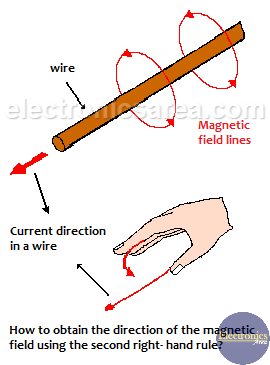Second Right-Hand Rule – Electromagnetism

Magnetic field created by a current in a wire.

When an alternating current or direct current flows through a wire, a non-visible effect called the electromagnetic field is generated around it. This field forms circles around the cable as shown in the figure. There are circles near and far from the wire simultaneously.

The magnetic field is more intense the closer it is to the wire and this intensity decreases as it moves away from it until its effect is zero.

The magnetic flux direction can be found if the direction of the current in the conductor wire is known and the Second Right-Hand Rule is used. (see the figure).This effect is very easy to visualize in direct current. The formula to obtain the magnetic field in a long conducting wire is: B = u.I / (2.p.d), where:

• B: magnetic field
• u: is the air permeability
• I: current through the cable
• p: Pi = 3.1416
• d: distance from the cable

This formula is valid as long as “d” is much smaller than the length of the wire.

Second right-hand rule applied to a wire wrapped in the shape of a coil

If there is a wire of N turns, the resulting magnetic field would be: B = N.m.I / (2.r).

The magnetic field at the center of a wire of N turns is: B = N.m.I / (2.r), where “r” is the radius of a turn.

The above formula is valid only when the radius of the turn is larger than the length of the coil. When the opposite occurs, B has the formula: B = u . n . I, where:

• n = N/L
• N = total number of turns
• L = coil length

It is important to know that:

– A current in a wire generates a magnetic field and …
– A magnetic field near a wire generates a current.

The best known applications, of a magnetic field created by a current in a wire, use alternating current. For example:

– Inductors: Where energy is stored as a magnetic field.
– Transformers: Where the alternating current generates an alternating magnetic field in the primary winding that induces, in the secondary winding, another magnetic field that in turn generates a current at the transformer output.

• 20
•
•
•
•
•
•
•
•
20
Shares
•
20
Shares
• 20
•
•
•
•
•
•
•